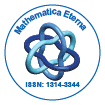# Mathematica EternaOpen Access

ISSN: 1314-3344

## Abstract

### Surfaces of Arbitrary Constant Negative Gaussian Curvature and Related Sine-Gordon Equations

Paul Bracken

Surfaces of arbitrary constant negative Gaussian curvature are in-vestigated using the fundamental equations of surface theory and the notion of line congruences. It is shown that such surfaces can be generated by means of solutions to a particular form of sine-Gordon equation.A Backlund transformation is found for this equation and it is shown how this can be used to construct nontrivial solutions to it. The theorem of permutability is formulated for the system as well.

Top